# Data Conversion Transformation in SSIS 2008R2 Example

0
17509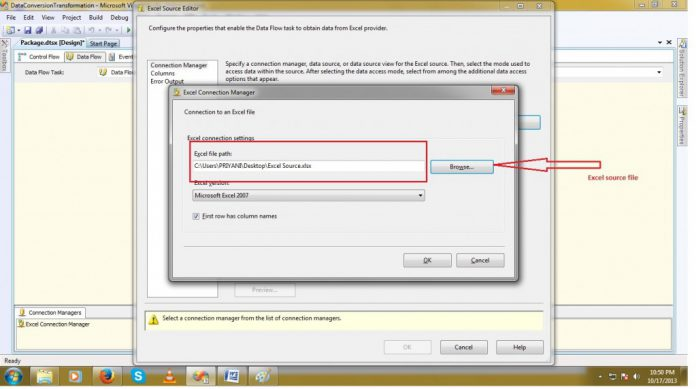Friends,

The data conversion transformation takes input columns and creates a copy of that column with selected (new) data type. If source columns data types are not matched with target columns, some cases SSIS will throw validation/failure error.

SQL Server to SSIS data type:

 SQL server SSIS smallint two-byte signed intger (DT_I2) int four-byte signed integer (DT_I4) bigint eight-byte signed integer (DT_I8) float double-precision float (DT_R8) char,varchar string (DT_STR) nchar,nvarchar unicode string (DT_WSTR) decimal,numeric numeric(DT_NUMERIC) decimal decimal (DT_DECIMAL) smallmoney,money currency (DT_CY) date database date (DT_DBDATE) datetime database timestamp (DT_DBTIMESTAMP)

Now we gona discuss with the help of one simple package in SSIS. Let’s say we have two tables one is test master and second is subject master. Lets create a table called wrk_atnd where we put the attendance of guards

CREATE TABLE [wrk_atnd] (
[atnd_ou] float,
[atnd_rptno] nvarchar(255),
[atnd_emp_code] nvarchar(255),
[atnd_date] datetime,
[atnd_assign_no] nvarchar(255),
[atnd_pd_line_no] float,
[atnd_contract_no] nvarchar(255),
[atnd_cont_lineno] float,
[atnd_shift] nvarchar(255),
[atnd_data_type] nvarchar(255),
[atnd_reg_hours] float,
[atnd_ot_hors] float,
[atnd_site_id] nvarchar(255),
[atnd_status] nvarchar(255),
[atnd_post] nvarchar(255),
[atnd_wrk_rpt_no] nvarchar(255),
[atnd_remarks] nvarchar(255),
[atnd_from_time] nvarchar(255),
[atnd_to_time] nvarchar(255),
[OU] float
)

This table work as a destination table where the excel data will be loaded. Let’s take a excel source file and move the excel data into sql server table.
Now configure the excel source file.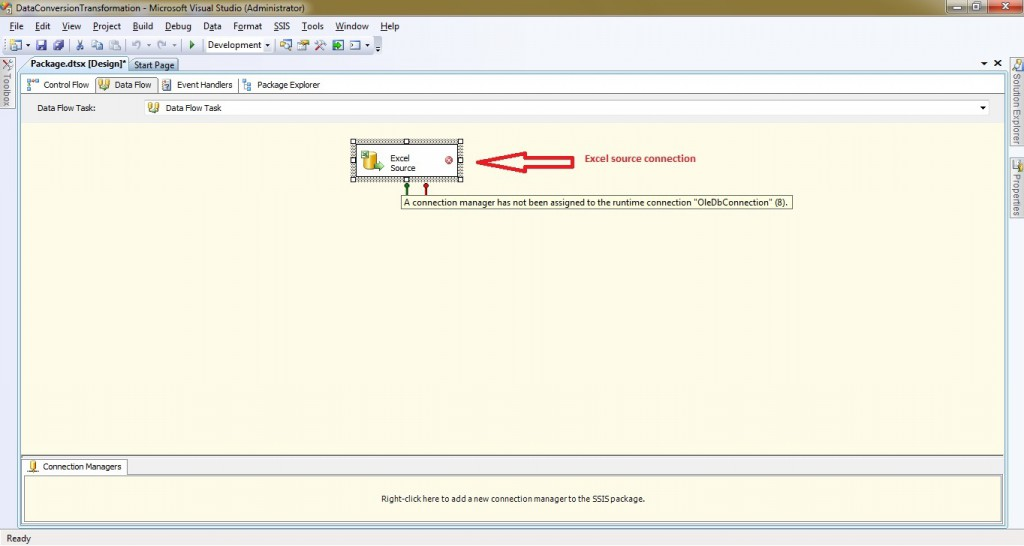Now add the data conversion transformation.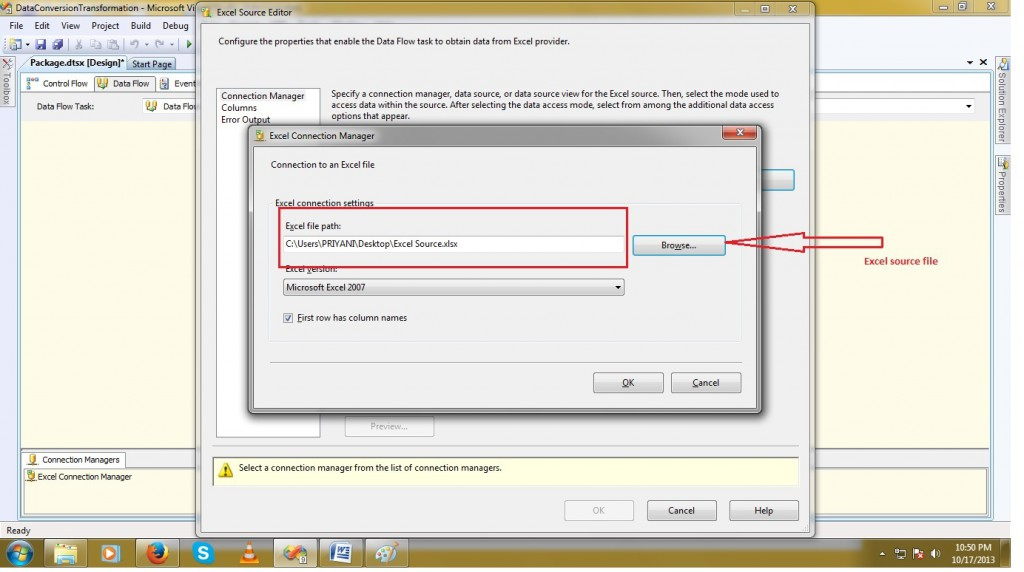To configure the data conversion double clicks on Transformation.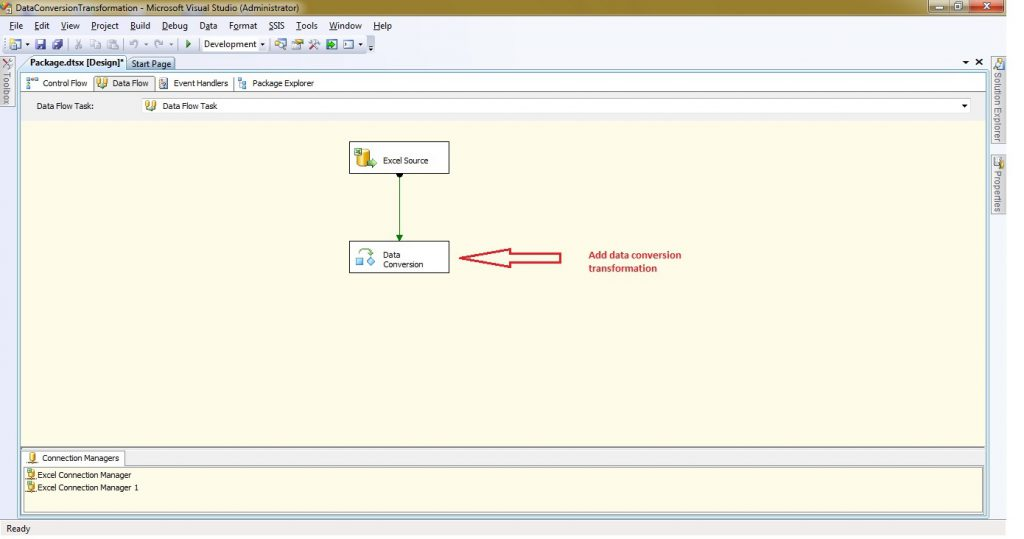Check the available input columns for which the data type will be changed. We can alias the input columns also. Select the compatible data type in Data type column and click on OK. Now we gona take the OLEDB Destination for moving the excel source data into table.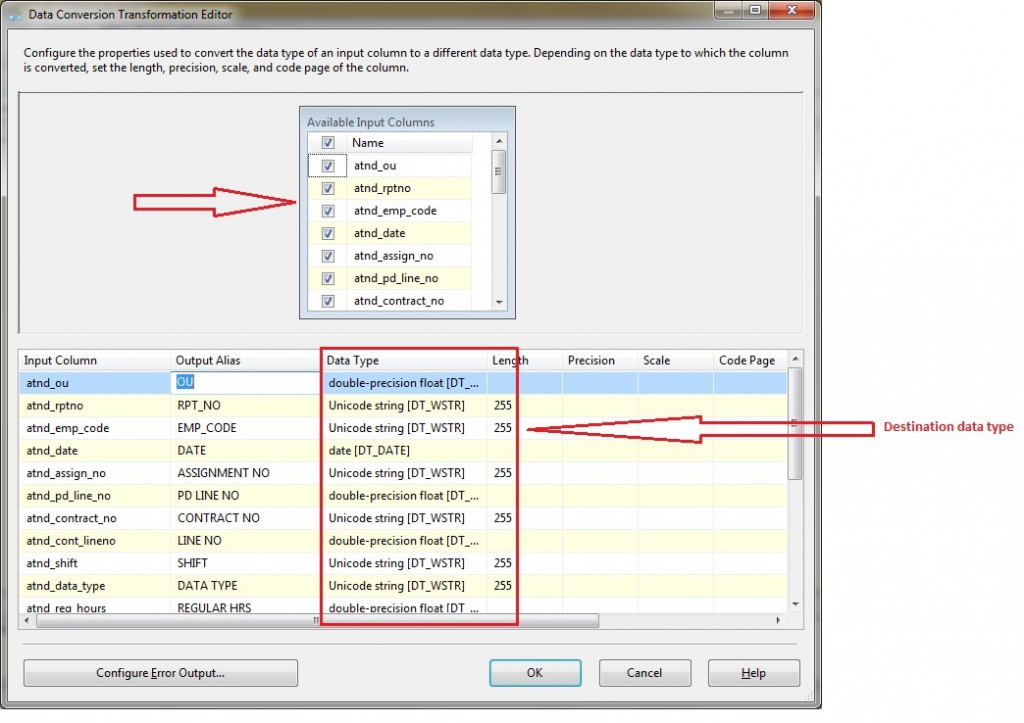For checking the data we have added the data viewer.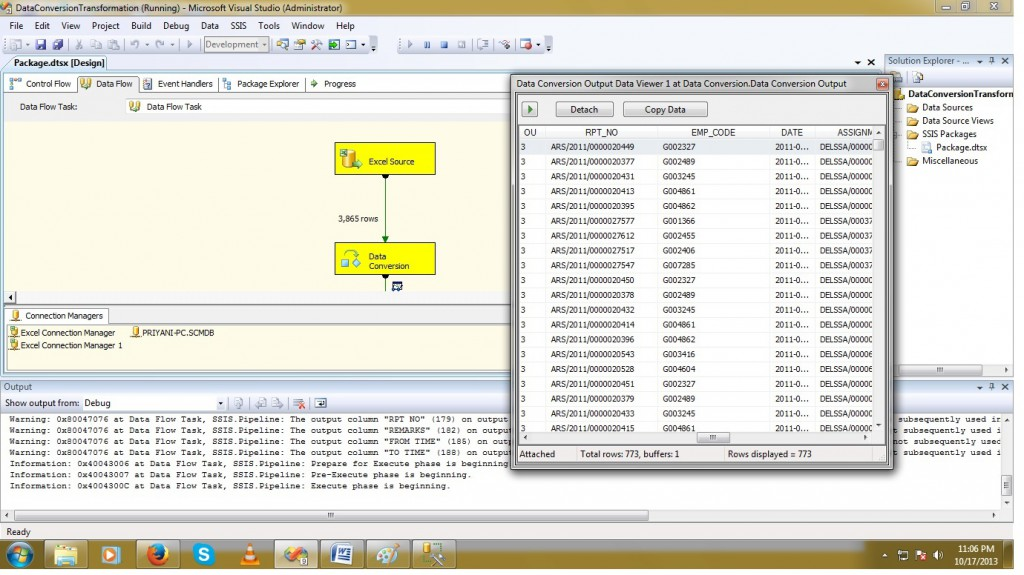Now Execute the package and see the result.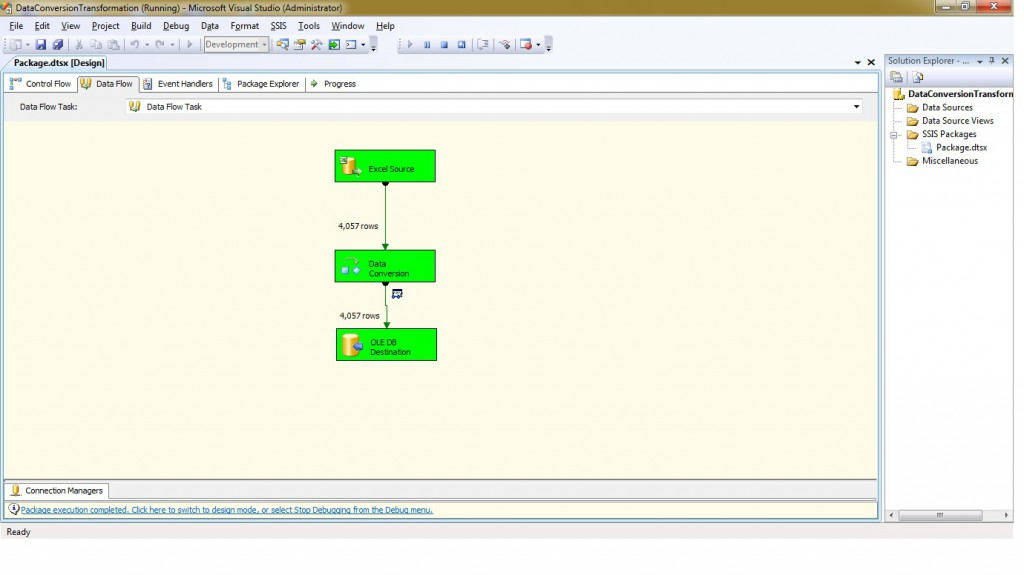You can see the data is inserted to the database table and we changed the datatypes using data conversion transformation while moving from Source to Destination. Give a try guys !! Thanks Sushil for the doc.

Regards,
Roopesh Babu V